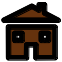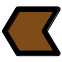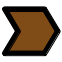# Finite group software

## What is group?

group is a small software intented to illustrate the theory of finite groups. This is a UNIX command (or DOS for Windows) which must be ran from any shell window. Give your operation table and group will test it for you and will return all subgroups with their properties. That's all! group is a free software distributed under the GNU general public licence. You can freely download, use, modify and re-distribute it.

## Goup theory

Definition of group

A group is a set G in which an operation has been defined (noted * by convention). The couple (G,*) must verify the following axioms:
(A1) Closure: For any a,b in G a*b belongs to G
(A2) Associativity: For any a,b,c in G then (a*b)*c=a*(b*c)
(A3) Unity: It exists a unit element noted e such that e*a=a*e=a for any a in G
(A4) Inversibility: For any a in G, it exists an element noted a-1 (the inverse of a) such that a*a-1=a-1*a=e

Abelian group

A group (G,*) is an Abelian group if the commutativity axiom is verified:
(A5) Commutativity: For any a,b in G then a*b=b*a

Subgroup

A subgroup H is a non-empty subset of G such that: For any a,b in H, a*b-1 belongs to H
A subgroup is a group for the operation *

Normal subgroup

A subgroup H of G is a normal subgroup if: For any a in G and for any h in H, a*h*a-1 belongs to H

Indeed, the mathematical theory of groups is much larger than the above summary. It exists many extended books and internet sites in which you can find all details and comments on the theory. But, we have limited the summary to the only definitions implemented in the software group.

## Example

Here is an example of use of group. In books of mathematics devoted theory of groups; first exercices on groups are sometimes based on the explicit construction of groups by giving algebraic relationships on elements. For example,

``` Construct a group generated by two elements i and j such as i2=e j2=e ij=ji and find all subgroups and normal subgroups. ```

What do we have to do? The first step is to construct the operation table of the group by applying the algebraic relationships. We get,

 * e i j ij e e i j ij i i e ij j j j ij e i ij ij j i e

The problem is now to check if axioms (A1) - (A5) apply. Indeed, for such an example, it can be done by hands. But you can imagine that the exercice becomes tedious (especially to check associativity) for larger groups. The other solution is to write a text file named for instance `exercice.txt`

``` # Input file to solve my exercice ORDER=4 SET=e i j ij OPERATION=+ TABLE= e i j ij i e ij j j ij e i ij j i e ```

This is the imput file for group. It contains four fields which are,
- `ORDER=n` the number `n` of elements (order of the group)
- `SET=a1 ... an` a list of symbols for elements (3 characters maximum per element)
- `OPERATION=*` a one character symbol (*, +, o or other) for the operation
- `TABLE=ai*aj` the operation table of the group. This is a table of `n2` entries where `i` and `j` runs from 1 to `n`.

Then run group by typing
`group exercice.txt`
in a command window. The output is,

``` Operation table * e i j ij e e i j ij i i e ij j j j ij e i ij ij j i e Inverse table element e i j ij inverse e i j ij The set is an Abelian group List of subgroups {e} NORMAL {e,i} NORMAL {e,j} NORMAL {e,ij} NORMAL {e,i,j,ij} NORMAL ```

You can observe that all elements of the group are the product of elements of normal subgroups {e,i} and {e,j} which are cyclic of order 2 and whose intersection is {e}. The group is therefore Z/2Z X Z/2Z that is Klein's group.

### History of releases

10 january 2010, release 0.1
- Check group axioms of any operation table.
- Find subgroups.
- Find normal subgroups.

### Compiling group

To compile the source of group on Unix/Linux systems, download the tarball group-0.1.tar.gz and untar it with
`tar -zxvf group-0.1.tar.gz`
then enter in the directory group-0.1
`cd group-0.1`
configure the makefile with
`./configure`
compile the source
`make`
finally install the package (under root)
`make install`
It should well work. Complete instructions for installation are available in the file INSTALL.

## Contact

Group is developed by Alain Le Bot . But other developers are welcome.

Bug report and requirements to alain.le-bot@ec-lyon.fr

Copyright (C) 2010 Alain Le Bot, Last revision 30th april 2010